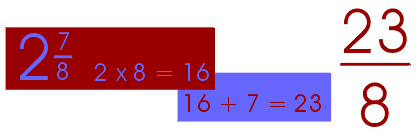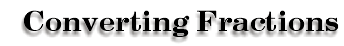One (1) can take the form of a fraction. This property will be important when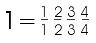you add or subtract fractions with unlike denominators.

 Any whole number can be converted to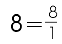a fraction by simply placing it over ONE.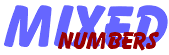A whole number combined with a fraction creates a mixed number.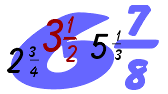To convert an improper fraction into a mixed number, divide the numerator by the denominator and use the remainder as the new numerator. The denominator stays the same.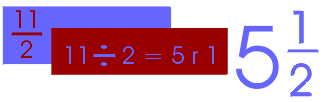To convert a mixed number to a fraction, multiply the whole number by the denominator and add the answer to the numerator. This new number becomes the new numerator. Keep the original denominator.